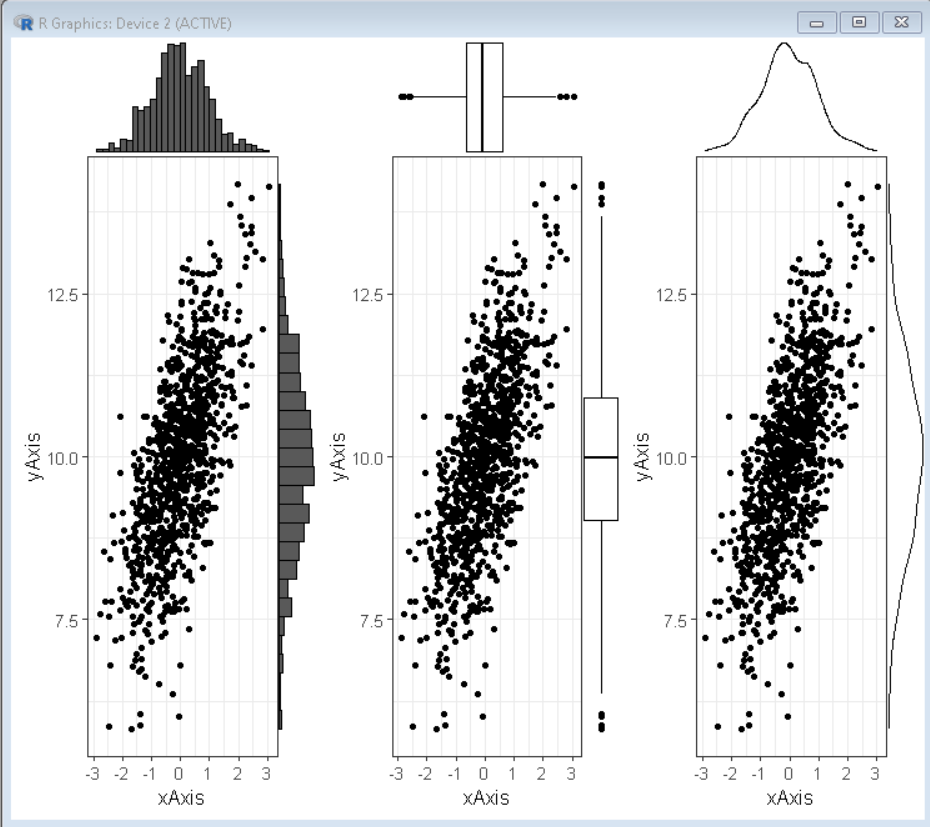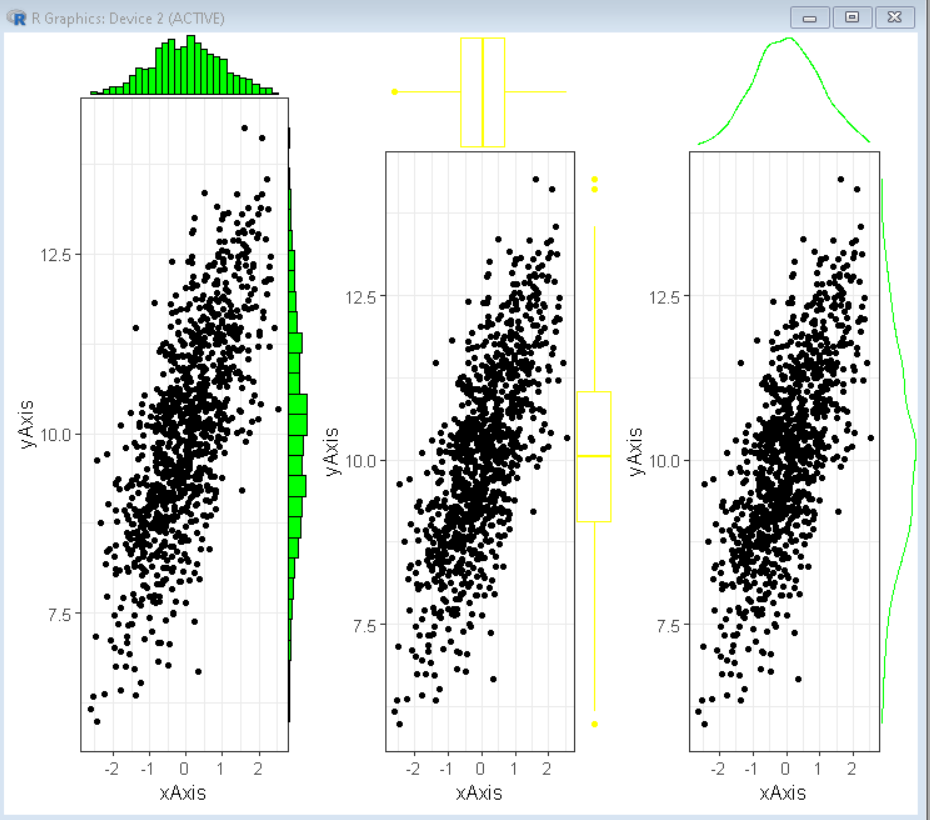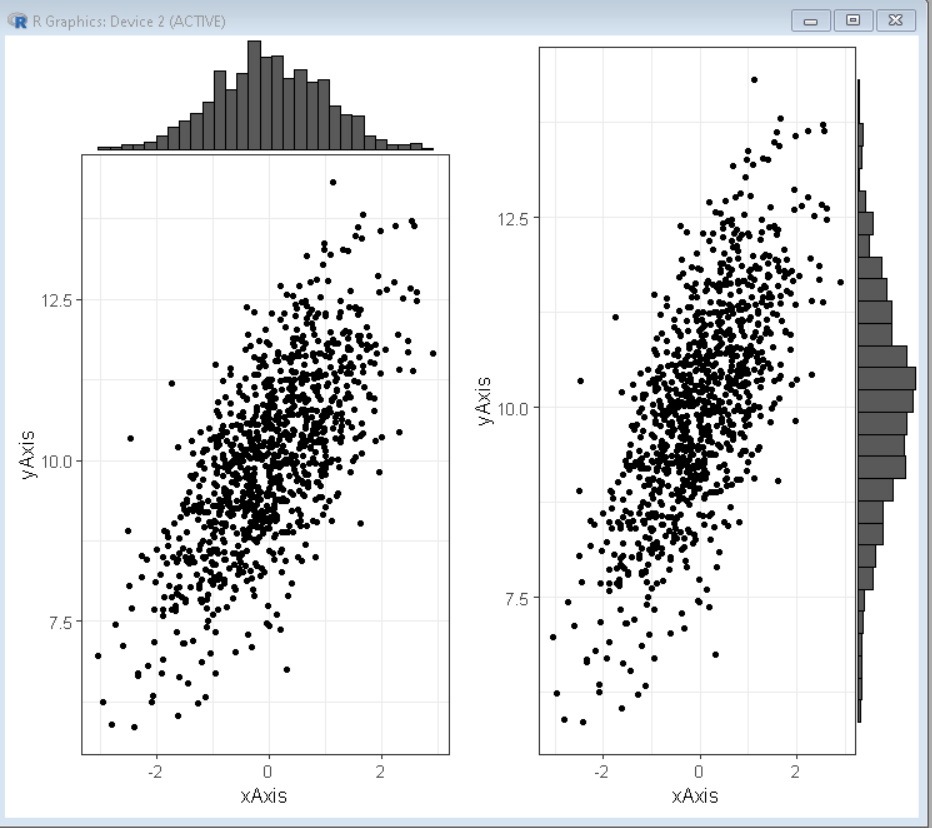R ggplot2 – Marginal Plots

• Last Updated : 16 Nov, 2021

A marginal plot is a scatterplot that has histograms, boxplots, or dot plots in the margins of the x- and y-axes. It allows studying the relationship between 2 numeric variables. The base plot visualizes the correlation between the x and y axes variables. It is usually a scatterplot or a density plot. The marginal charts are commonly plotted on the top and right margin of the base plot and they show the distribution of x and y axes variables using a histogram, barplot, or density plot. This helps us to visualize the distribution intensity at different values of variables along both axes.

To plot a marginal plot in the R  Language, we will use the ggExtra package of the R Language. The ggExtra is a collection of functions and layers to enhance ggplot2. The ggMarginal() function can be used to add marginal histograms/boxplots/density plots to ggplot2 scatterplots.

Installation:

To install the ggExtra package we use:

install.packages("ggExtra")

Creation of Marginal plots

To create marginal plots we use the following function to make a marginal histogram with a scatter plot.

Syntax: ggMarginal( plot, type )

Parameters:

• plot: Determines the base scatter plot over which marginal plot has to be added.
• type: Determines the type of marginal plot i.e. histogram, boxplot and density.

Example: Basic scatter plot with marginal histogram, density plot, and box plot all arranged on a page using the grid.arrange() function of ggExtra package.

R

 # load library tidyverse, gridExtra and ggExtralibrary(tidyverse)library(ggExtra)library(gridExtra) # set themetheme_set(theme_bw(12)) # create x and y vectorxAxis <- rnorm(1000)                yAxis <- rnorm(1000) + xAxis + 10  # create sample data framesample_data <- data.frame(xAxis, yAxis) # create scatter plot using ggplot() functionplot <- ggplot(sample_data, aes(x=xAxis, y=yAxis))+          geom_point()+        theme(legend.position="none") # use ggMarginal function to create# marginal histogram, boxplot and density plotplot1 <- ggMarginal(plot, type="histogram")plot2 <- ggMarginal(plot, type="boxplot")plot3 <- ggMarginal(plot, type="density") # combine plots in a gridgrid.arrange( plot1, plot2, plot3, ncol=3)

Output:Color and size customization

We can customize the parameter of the ggmarginal() function to create the desired look for our marginal plot. We can use the size, fill, and color parameter of the ggmarginal() function to change the relative size, background fill color, and routine color of the marginal plot respectively.

Syntax: ggMarginal( plot, type, fill, color, size )

Parameters:

• plot: Determines the base scatter plot over which marginal plot has to be added.
• type: Determines the type of marginal plot i.e. histogram, boxplot, and density.
• fill: Determines the background fill color of the marginal plot
• color: Determines the outline color of the marginal plot
• size: determines the relative size of plot elements.

Example: Here, we have modified the plot from the above example with custom colors and size parameters.

R

 # load library tidyverse, gridExtra and ggExtralibrary(tidyverse)library(ggExtra)library(gridExtra) # set themetheme_set(theme_bw(12)) # create x and y vectorxAxis <- rnorm(1000)                yAxis <- rnorm(1000) + xAxis + 10  # create sample data framesample_data <- data.frame(xAxis, yAxis) # create scatter plot using ggplot() functionplot <- ggplot(sample_data, aes(x=xAxis, y=yAxis))+          geom_point()+        theme(legend.position="none") # use ggMarginal function to create# marginal histogram, boxplot and density plot# fill, colo and size property is used for customizationplot1 <- ggMarginal(plot, type="histogram", fill= "green", size=10)plot2 <- ggMarginal(plot, type="boxplot", color="yellow" )plot3 <- ggMarginal(plot, type="density", color="green") # combine plots in a gridgrid.arrange( plot1, plot2, plot3, ncol=3)

Output:Marginal plot for only one axis

Sometimes we need a marginal plot at only one axis either the x-axis or the y-axis. In that situation, we use the margins parameter of the ggmarginal() function. The axis where we want the marginal plot to appear is given as the value of argument margins.

Syntax: ggMarginal( plot, type, margins )

Parameters:

• plot: Determines the base scatter plot over which marginal plot has to be added.
• type: Determines the type of marginal plot i.e. histogram, boxplot, and density.
• margins: Determines the axis where the marginal plot is required

Example: Here, are two plots one with the marginal plot on the x-axis other on the y-axis.

R

 # load library tidyverse, gridExtra and ggExtralibrary(tidyverse)library(ggExtra)library(gridExtra) # set themetheme_set(theme_bw(12)) # create x and y vectorxAxis <- rnorm(1000)                yAxis <- rnorm(1000) + xAxis + 10  # create sample data framesample_data <- data.frame(xAxis, yAxis) # create scatter plot using ggplot() functionplot <- ggplot(sample_data, aes(x=xAxis, y=yAxis))+          geom_point()+        theme(legend.position="none") # use ggMarginal function to create# marginal histogram on x-axisplot1 <- ggMarginal(plot, type="histogram", margins='x') # use ggMarginal function to# create marginal histogram on y-axisplot2 <- ggMarginal(plot, type="histogram", margins='y') # combine plots in a gridgrid.arrange( plot1, plot2, ncol=2)

Output:My Personal Notes arrow_drop_up